# Chapter 3 Resistance Resistance of Conductors Resistance of

• Slides: 30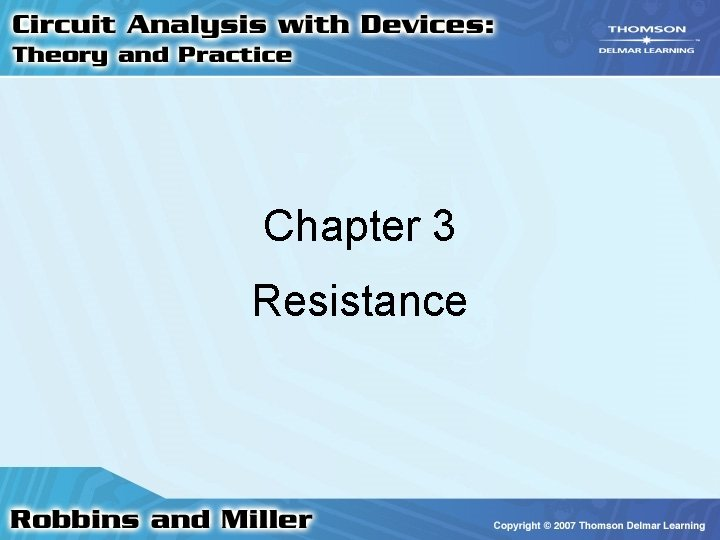Chapter 3 ResistanceResistance of Conductors • Resistance of material is dependent on several factors: – Type of Material – Length of the Conductor – Cross-sectional area – Temperature 2Type of Material • Atomic differences of materials cause variations in how electron collisions affect resistance • Differences produce resistivity 3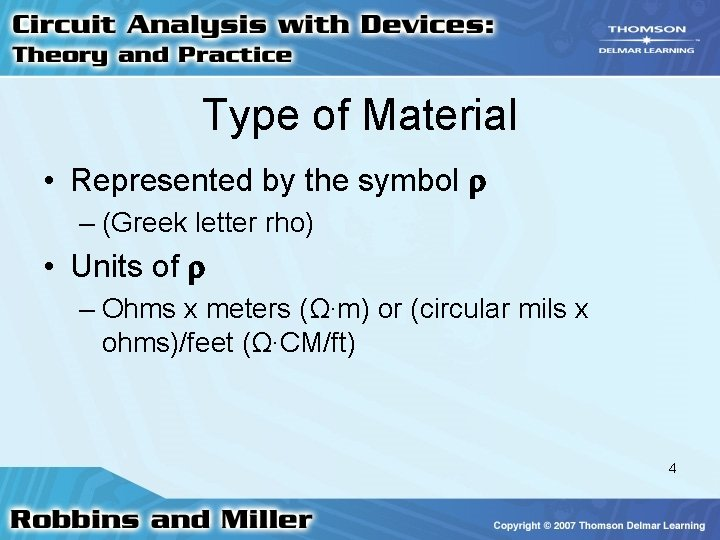Type of Material • Represented by the symbol – (Greek letter rho) • Units of – Ohms x meters (Ω∙m) or (circular mils x ohms)/feet (Ω∙CM/ft) 4Length • Resistance of a conductor – Directly proportional to its length – If you double the length of the wire, the resistance will double • = length – In meters or feet 5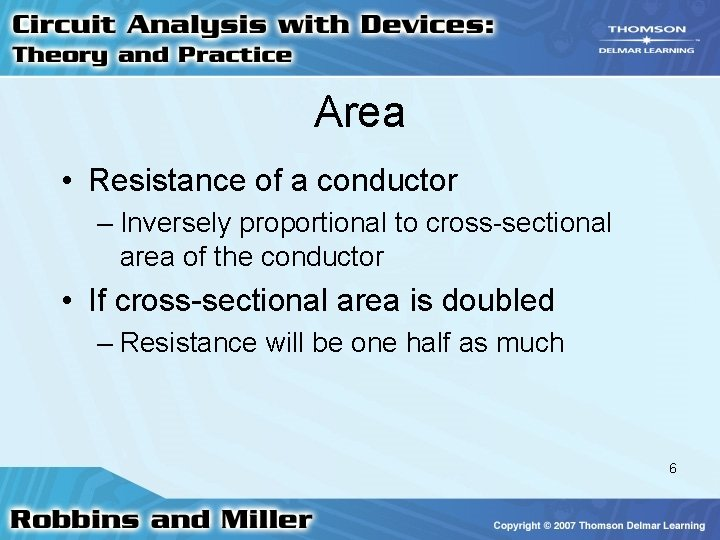Area • Resistance of a conductor – Inversely proportional to cross-sectional area of the conductor • If cross-sectional area is doubled – Resistance will be one half as much 6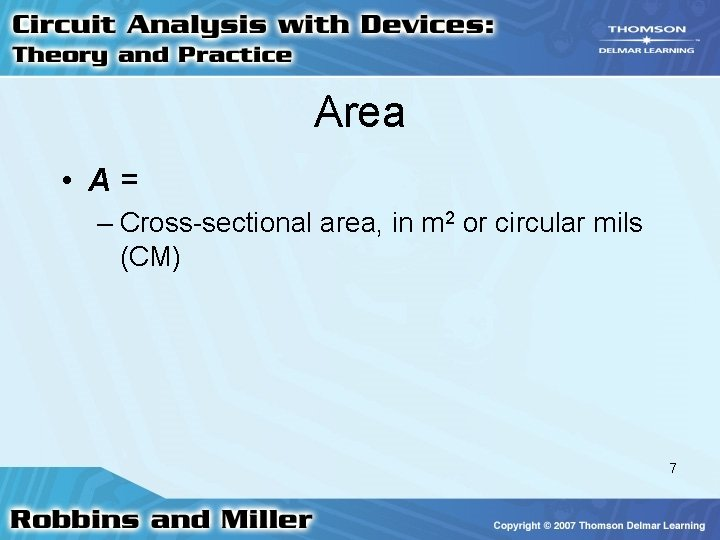Area • A= – Cross-sectional area, in m 2 or circular mils (CM) 7Resistance Formula • At a given temperature (usually 20 o C) • Formula can be used with both circular and rectangular conductors 8Electrical Wire Tables • American Wire Gauge is primary system to denote wire diameters • The higher the AWG number, the smaller the diameter 9Electrical Wire Tables • A given length of AWG 22 wire will have more resistance than the same length of AWG 14 wire • Larger gauge wires can handle more current 10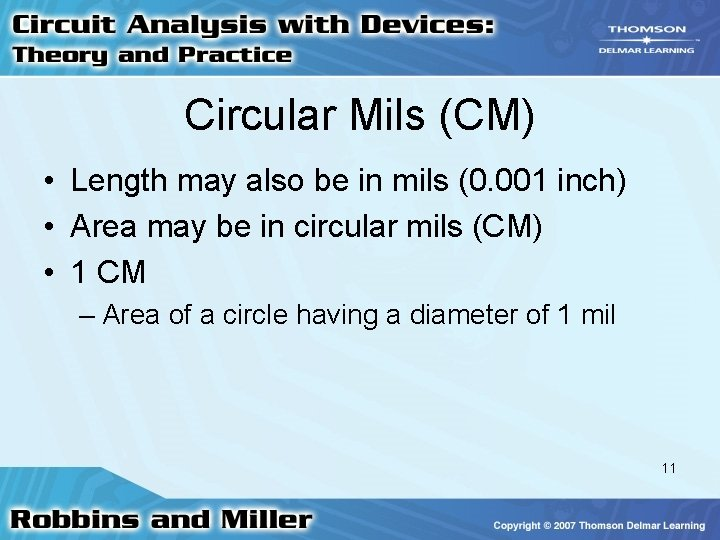Circular Mils (CM) • Length may also be in mils (0. 001 inch) • Area may be in circular mils (CM) • 1 CM – Area of a circle having a diameter of 1 mil 11Circular Mils (CM) • 1 square mil – Area of a square having sides of 1 mil • 1 CM = – /4 square mils 12Temperature Effects • For most conductors, a temperature increase causes an increase in resistance • Increase is relatively linear • In semiconductors and insulators – Increase in temperature results in decrease in resistance 13Temperature Effects • Any material for which the resistance increases with temperature is said to have a positive temperature coefficient • If it decreases, it has a negative coefficient 14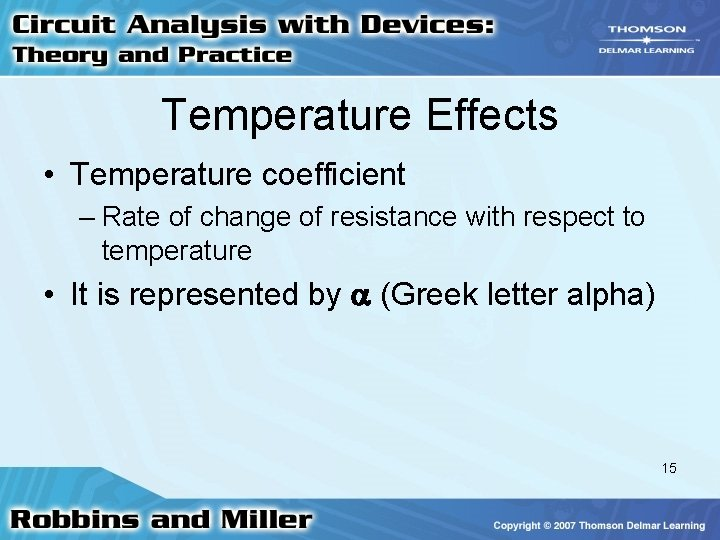Temperature Effects • Temperature coefficient – Rate of change of resistance with respect to temperature • It is represented by (Greek letter alpha) 15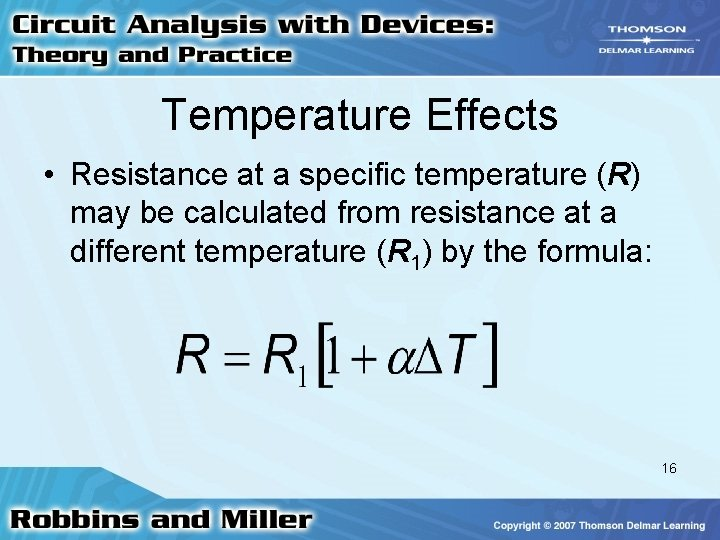Temperature Effects • Resistance at a specific temperature (R) may be calculated from resistance at a different temperature (R 1) by the formula: 16Temperature Effects • Where ΔT = – T 1 is the difference between the two temperatures in Celsius degrees 17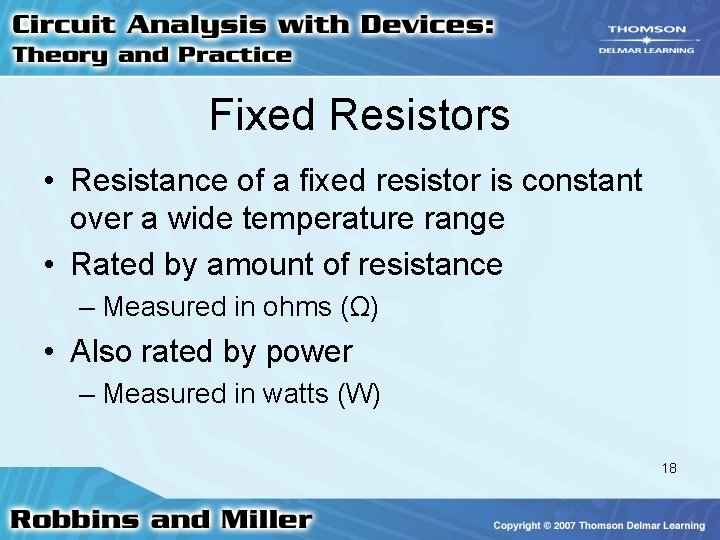Fixed Resistors • Resistance of a fixed resistor is constant over a wide temperature range • Rated by amount of resistance – Measured in ohms (Ω) • Also rated by power – Measured in watts (W) 18Fixed Resistors • Different resistors for different applications – Molded carbon composition – Carbon film – Metal Oxide – Wire-Wound – Integrated circuit packages 19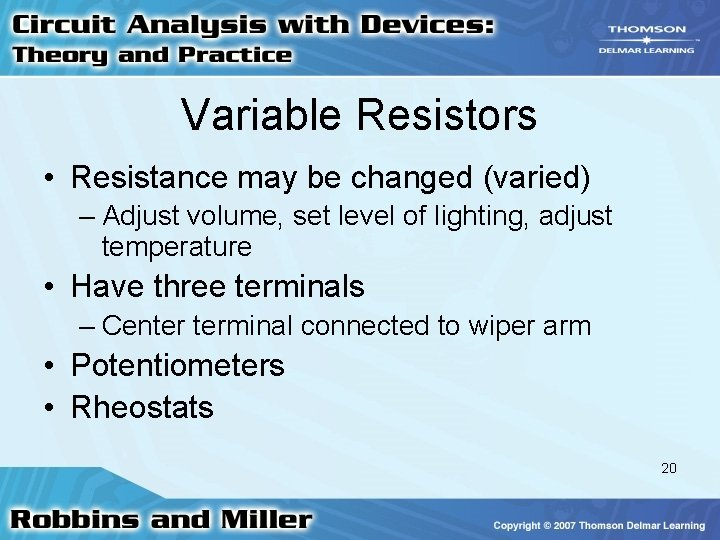Variable Resistors • Resistance may be changed (varied) – Adjust volume, set level of lighting, adjust temperature • Have three terminals – Center terminal connected to wiper arm • Potentiometers • Rheostats 20Color Code • Colored bands on a resistor provide a code for determining – Value – Tolerance – Reliability 21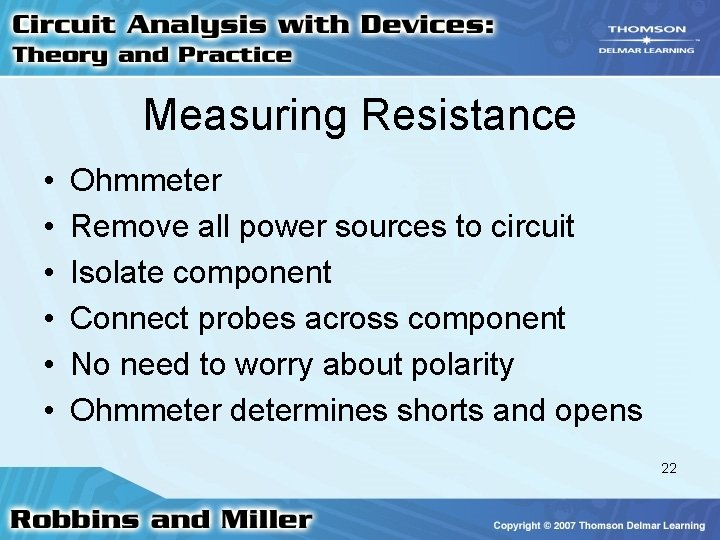Measuring Resistance • • • Ohmmeter Remove all power sources to circuit Isolate component Connect probes across component No need to worry about polarity Ohmmeter determines shorts and opens 22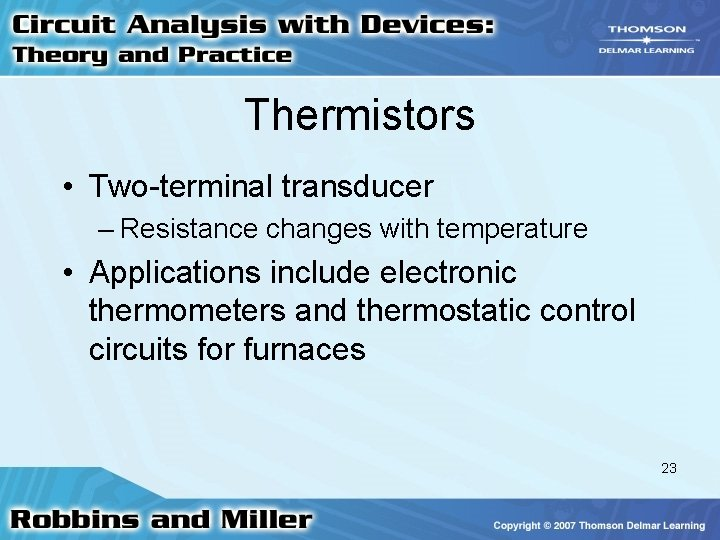Thermistors • Two-terminal transducer – Resistance changes with temperature • Applications include electronic thermometers and thermostatic control circuits for furnaces 23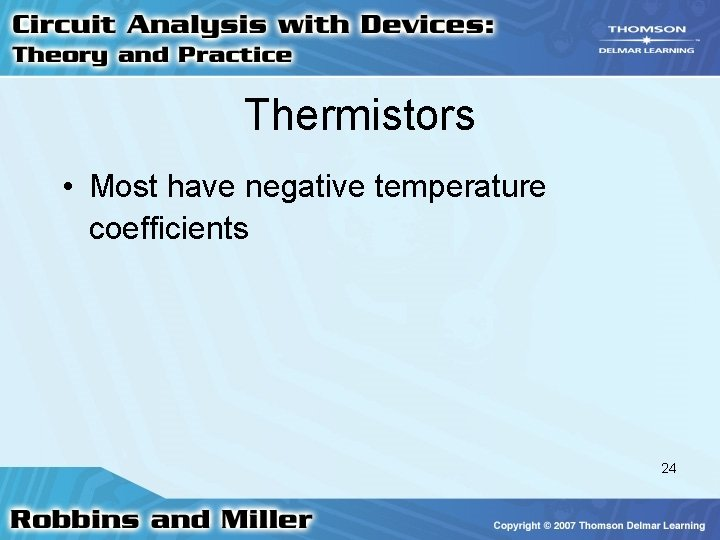Thermistors • Most have negative temperature coefficients 24Photoconductive Cells • Two-terminal transducers – Resistance determined by amount of light • May be used to measure light intensity or to control lighting • Used in security systems • Linear response (negative slope) 25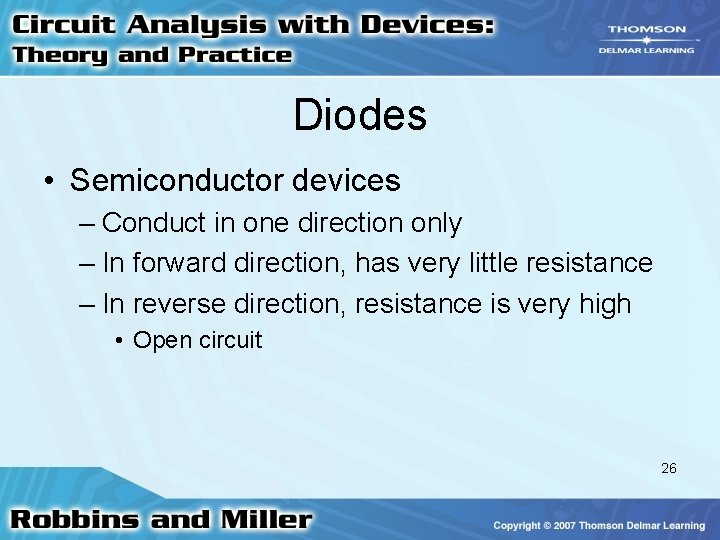Diodes • Semiconductor devices – Conduct in one direction only – In forward direction, has very little resistance – In reverse direction, resistance is very high • Open circuit 26Varistors • Resistors sensitive to voltage • High resistance when voltage is below breakdown value • Low resistance when voltage is above breakdown value • High power ratings – When used in surge protectors 27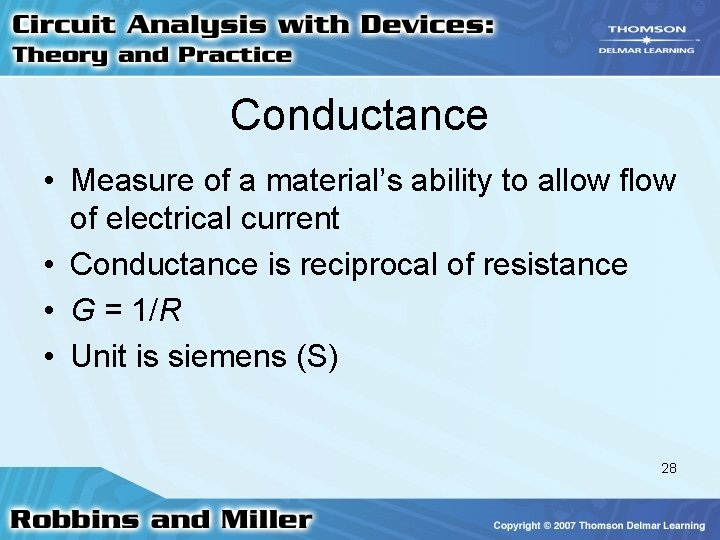Conductance • Measure of a material’s ability to allow flow of electrical current • Conductance is reciprocal of resistance • G = 1/R • Unit is siemens (S) 28Superconductors • Low temperatures – Resistance of some materials goes to almost zero • Temperature is called critical temperature 29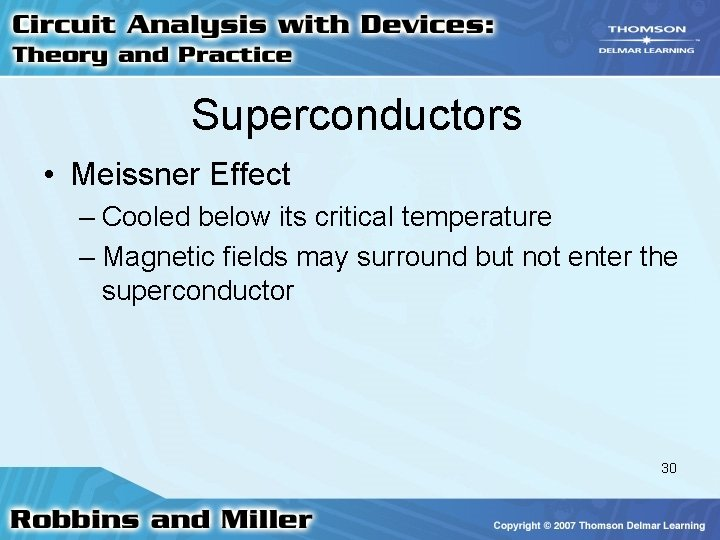Superconductors • Meissner Effect – Cooled below its critical temperature – Magnetic fields may surround but not enter the superconductor 30